Chapter 10.5, Problem 60EFinite Mathematics and Applied Cal...

7th Edition
Stefan Waner + 1 other
ISBN: 9781337274203

Solutions

Chapter
SectionFinite Mathematics and Applied Cal...

7th Edition
Stefan Waner + 1 other
ISBN: 9781337274203
Textbook Problem

In Exercises 59–64, match the graph of f to the graph of f ′ . (The graphs of f ' are shown after Exercise 64.)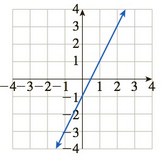Graph of derivatives for Exercises 59-64:(A)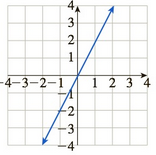(B)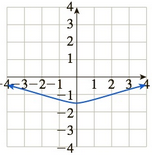(C)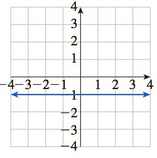(D)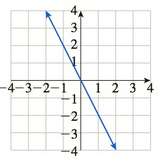(E)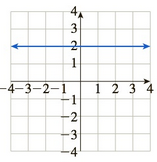(F)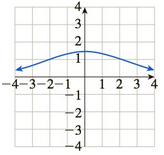To determine

The graph of f of f from the provided graphs.

The graph of f is:The graphs provided for f are: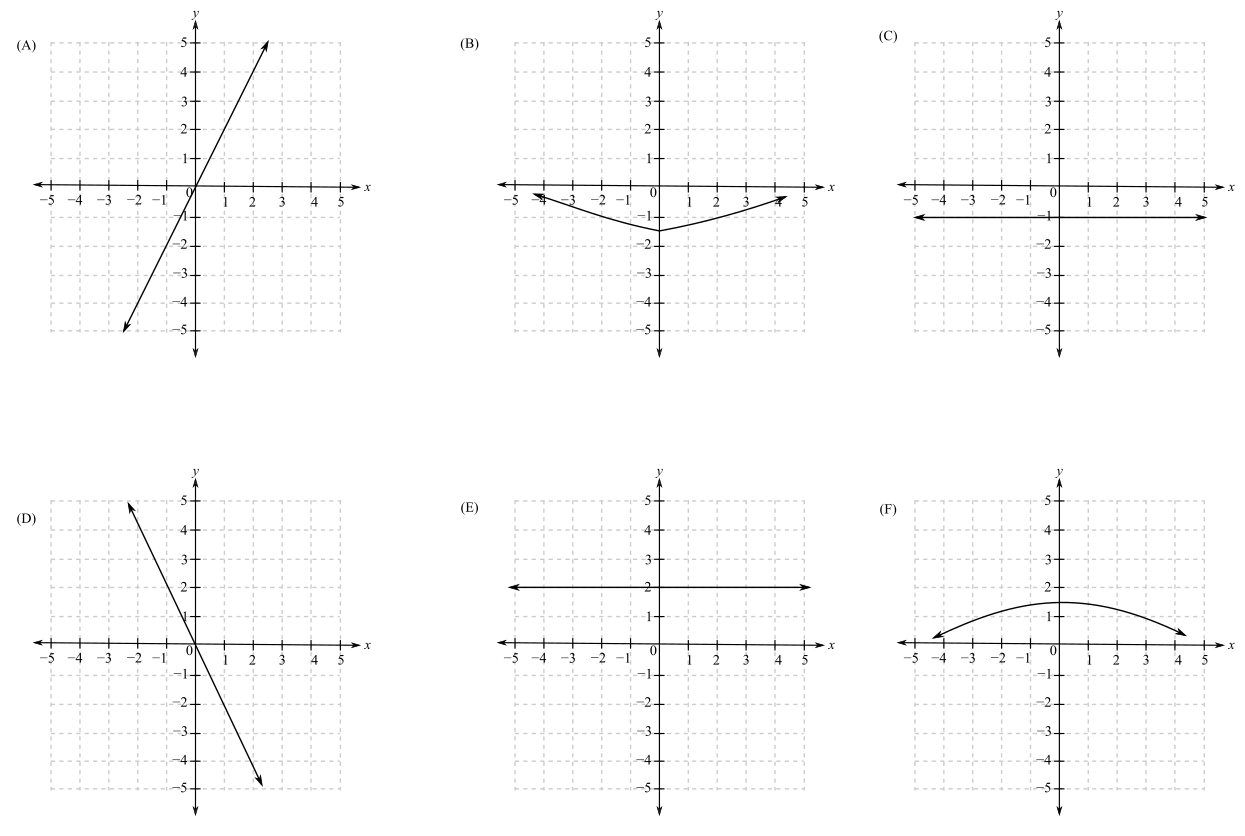Explanation

Given Information:

The graph of f is:

The graphs provided for f are:

When a function is increasing or rising the slope of the function is positive and greatest where the tangent is more slanted whereas when the function is decreasing or falling its slope is negative and it has the least value where the tangent is the most slanted.

Now, from the graph, it can be seen that it is a straight line and rising.

Thus, the slope of the straight line is m=y2y1x2x1

From the graph, x1=1,

Still sussing out bartleby?

Check out a sample textbook solution.

See a sample solution

The Solution to Your Study Problems

Bartleby provides explanations to thousands of textbook problems written by our experts, many with advanced degrees!

Get Started